### Home > GC > Chapter 7 > Lesson 7.2.5 > Problem7-87

7-87.

For each pair of triangles below, determine if the triangles are congruent. If the triangles are congruent,

• complete the correspondence statement,

• state the congruence property,

• and record any other ideas you use that make your conclusion true.

Otherwise, explain why you cannot conclude that the triangles are congruent. Note that the figures are not necessarily drawn to scale.

1. $ΔABC≅\Delta\underline{\qquad}$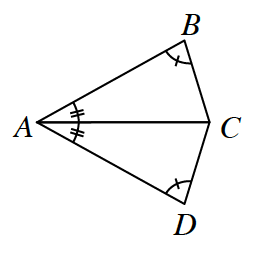$ΔABC≅\Delta{\underline{\ \ {ADC}\ }}$
$\text{AAS}≅$
($AC = CA$ Reflexive helps)

1. $ΔSQP ≅ Δ\underline{\qquad}$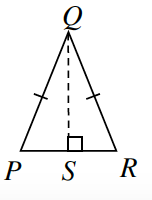$ΔSQP ≅ Δ\underline{\ \ SQR\ }$
$\text{HL} ≅$
($QS = SQ$ Reflexive helps)

1. $ΔPLM ≅ Δ\underline{\qquad}$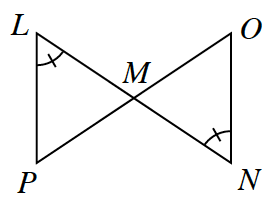No solution: $∠L≅∠N$ and $∠LMP≅∠NMO$, but only enough information to prove similar by $\text{AA}\sim$.

1. $ΔWXY ≅ Δ\underline{\qquad}$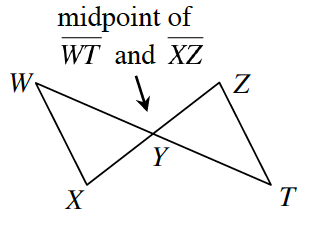• $ΔWXY ≅ Δ\underline{\ \ TZY\ }$
$\text{SAS}≅$
($WY=TY$ & $XY=ZY$ by definition of midpoint; $∠WYX=∠TYZ$ by vertical angles)

1. $ΔEDG ≅ Δ\underline{\qquad}$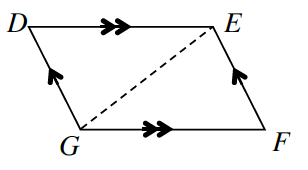• $ΔEDG ≅ Δ\underline{\ \ GFE\ }$ you figure out why, add extra information.

1. $ΔABC ≅ Δ\underline{\qquad}$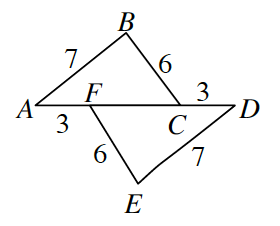• $ΔABC ≅ Δ\underline{\ \ DEF\ }$ you figure out why, add extra information.